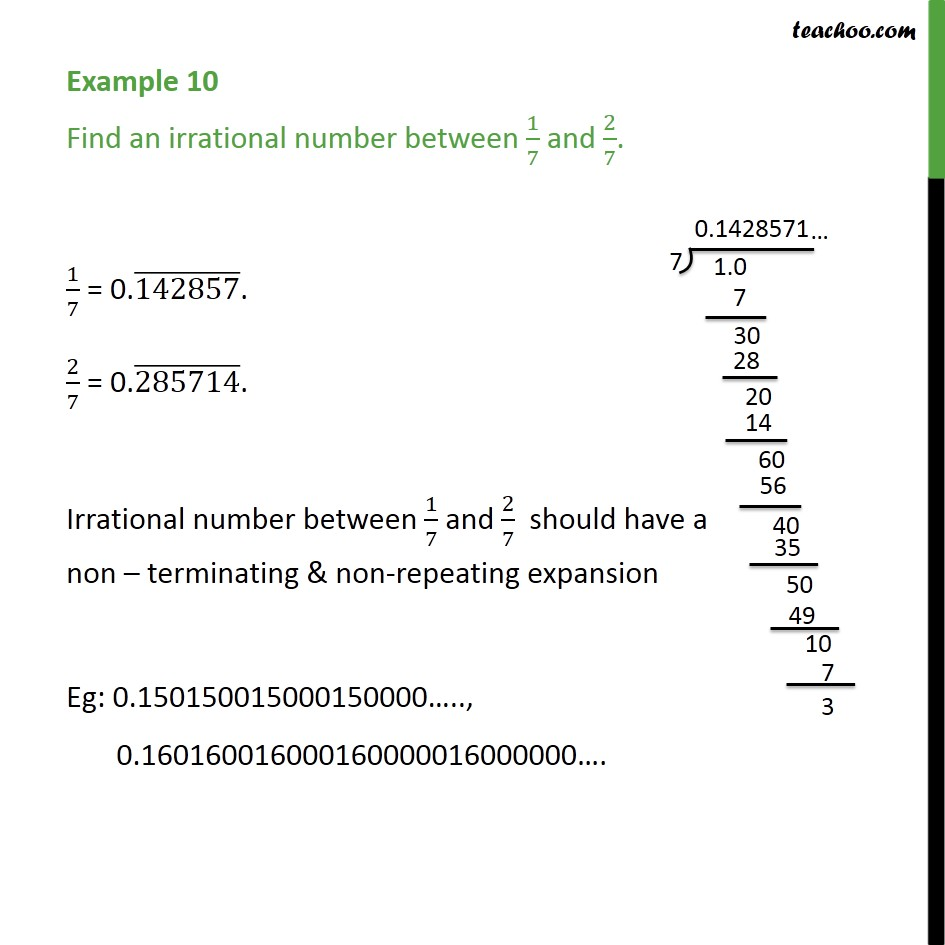Learn All Concepts of Chapter 1 Class 9 - FREE. Check - Chapter 1 Class 9 Maths1. Chapter 1 Class 9 Number Systems
2. Concept wise
3. Finding irrational numbers b/w two numbers

Transcript

Example 10 Find an irrational number between 1/7 and 2/7. 1/7 = 0.(142857) ̅. 2/7 = 0.(285714) ̅. Irrational number between 1/7 and 2/7 should have a non – terminating & non-repeating expansion Eg: 0.150150015000150000….., 0.160160016000160000016000000….

Finding irrational numbers b/w two numbers